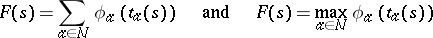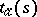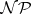# Scheduling theory

(diff) ← Older revision | Latest revision (diff) | Newer revision → (diff)

A branch of applied mathematics (a division of operations research) concerned with mathematical formulations and solution methods of problems of optimal ordering and coordination in time of certain operations. Scheduling theory includes questions on the development of optimal schedules (Gantt charts, graphs) for performing finite (or repetitive) sets of operations. The area of application of results in scheduling theory include management, production, transportation, computer systems, construction, etc.

The problems that scheduling theory deals with are usually formulated as optimization problems for a process of processing a finite set of jobs in a system with limited resources. A finite set of jobs is what distinguishes scheduling models from similar models in queueing theory, where basically infinite flows of activities are considered. In all other respects the starting points of the two theories are close. In scheduling theory, the time of arrival for every job into the system is specified. Within the system the job has to pass several processing stages, depending on the conditions of the problem. For every stage, feasible sets of resources are given, as well as the processing time depending on the resources used. The possibility of interruptions in the processing of certain jobs (so-called pre-emptions) can also be stipulated. Constraints on the processing sequence are usually described by a transitive anti-reflexive binary relation. Algorithms for the evaluation of characteristics of large partially ordered sets of jobs constitute the essence of the part of scheduling theory called network analysis (cf. Network model; Network planning). Sometimes, in scheduling models durations of re-adjustments are specified that are necessary when one job in process is replaced by another, as well as certain other conditions.

It should be mentioned that, being practically oriented, scheduling theory does not seek to unify its terminology; along with the term "job" , terms like "task" , "operation" , etc., are also used. For the same reason a processing schedule is formally defined in several ways. In the general case, a schedule can be understood as a single-valued mapping that to every job at every moment assigns a certain set of resources. As criteria, total and maximal costs of the formare usually taken, whereis the completion time of jobaccording to schedule,is the set of all jobs and theare non-decreasing functions, called cost functions. In practical formulations the cost functions usually have a specific economical meaning (like freezing floating capital, damages by loss of customers, etc.). Multi-criterion problems are also considered.

In addition to deterministic models, stochastic models are also studied. In this case either the minimization problem for the expectation of one of the criteria used in a deterministic formulation is considered, or the probability of a certain event is minimized. Such an event can be, e.g., a delay in processing relative to described terms.

The basic approach to the solution of deterministic scheduling problems is the general algorithmic scheme of enumerative optimization (branch-and-bound). In this way most practical problems were successfully solved, and optimization procedures for the planning of jobs in time were developed (calendar planning). These have been realized in automatic control systems. Along with that, fast decision rules for a number of specific deterministic problems were obtained, necessary and sufficient conditions for their use were proved, and effective methods were proposed that are important for general discrete optimization problems (see ). Results from graph theory and mathematical programming are often applicable to the development of optimization algorithms of this kind. Work on-completeness published at the beginning of the 1970's stimulated numerous studies on the complexity of scheduling problems (see, e.g., ). The results of this research enhanced the interest in approximation algorithms and bounds on their performance (see, e.g., ). Scheduling presents easily formulated and practically meaningful problems, which are "touchstones" for many approaches and methods of discrete optimization.

How to Cite This Entry:
Scheduling theory. Encyclopedia of Mathematics. URL: http://encyclopediaofmath.org/index.php?title=Scheduling_theory&oldid=17952
This article was adapted from an original article by Ya.B. ZinderV.V. Shkurba (originator), which appeared in Encyclopedia of Mathematics - ISBN 1402006098. See original article